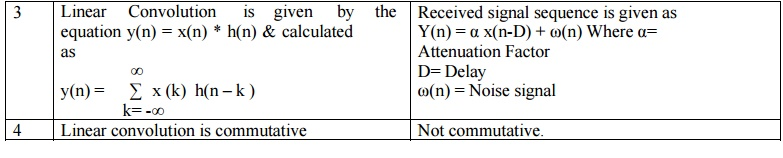Home | | Discrete Time Systems and Signal Processing | Difference Between Linear Convolution and Correlation

# Difference Between Linear Convolution and Correlation

Linear Convolution: In case of convolution two signal sequences input signal and impulse response given by the same system is calculated

DIFFERENCE BETWEEN LINEAR CONVOLUTION AND CORRELATIONLinear Convolution

1. In case of convolution two signal sequences input signal and impulse response given by the same system is calculated

2. Our main aim is to calculate the response given by the system.

3. Linear Convolution is given by the equation y(n) = x(n) * h(n) & calculated as4. Linear convolution is commutative

Correlation

1. In case of Correlation, two signal sequences are just compared.

2. Our main aim is to measure the degree to which two signals are similar and thus to extract some information that depends to a large extent on the application

3. Received signal sequence is given as Y(n) = α x(n-D) + ω(n) Where α= Attenuation Factor D= Delay ω(n) = Noise signal

4. Not commutative.

Study Material, Lecturing Notes, Assignment, Reference, Wiki description explanation, brief detail
Digital Signal Processing : Signals and System : Difference Between Linear Convolution and Correlation |